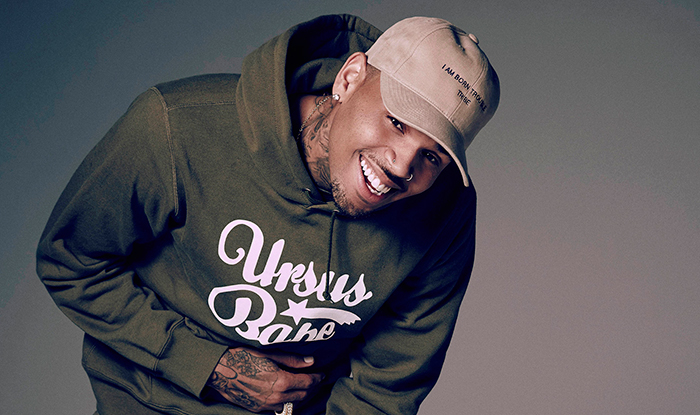“Zero”

I thought we were great
You took your love back and ran us off track
I counted all the days
‘Til you would come back. How stupid was that?

Now you’re missing what we used to have
Guess the vodka brought the feeling back
I was caught up in the aftermath
But now, if you really want to know

Ask how many nights I’ve been thinking of you, zero, zero
Gave a hundred percent but all I got from you, zero, zero
I thought you were the one, then you turned around
Found somebody better, like I never met ya
Ask how many nights I’ve been thinking of you, zero, zero
(zero, zero, zero, zero, zero, zero, zero, zero, zero, zero, zero)
That’s how many fucks I give

Wake up with a dime
Get up to no good better than you could
Go out, free our minds
Like you never would, never understood

Now you’re missing what we used to have
You been creeping round my Instagram
I was caught up in the aftermath
But now, if you really wanna know

Ask how many nights I’ve been thinking of you, zero, zero
Gave a hundred percent but all I got from you, zero, zero
I thought you were the one, then you turned around
Found somebody better, like I never met ya
Ask how many nights I’ve been thinking of you, zero, zero
(zero, zero, zero, zero, zero, zero, zero, zero, zero, zero, zero)
That’s how many fucks I give

Broke back then but a nigga rich now
Got dollars, got euros, got yen, I got pounds
Zeros, zeros
Calling me up cause your bank check bounced
My new chick one hundred
And she got her own dinero, zero

Ask how many nights I’ve been thinking of you, zero, zero
Gave a hundred percent but all I got from you, zero, zero
I thought you were the one, then you turned around
Found somebody better, like I never met ya
Ask how many nights I’ve been thinking of you, zero, zero
(zero, zero, zero, zero, zero, zero, zero, zero, zero, zero, zero, zero)
That’s how many fucks I give,
(zero, zero, zero, zero, zero, zero, zero, zero, zero, zero, zero)
That’s how many fucks I give